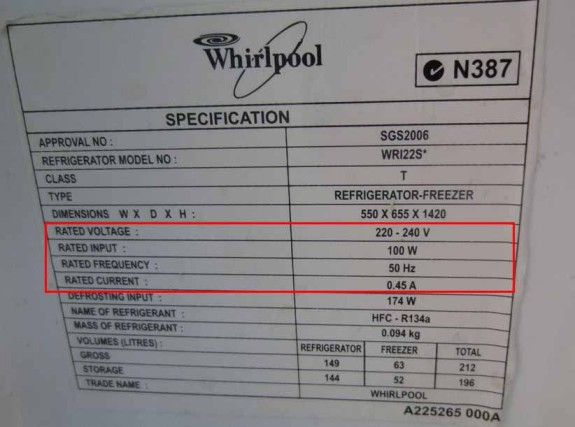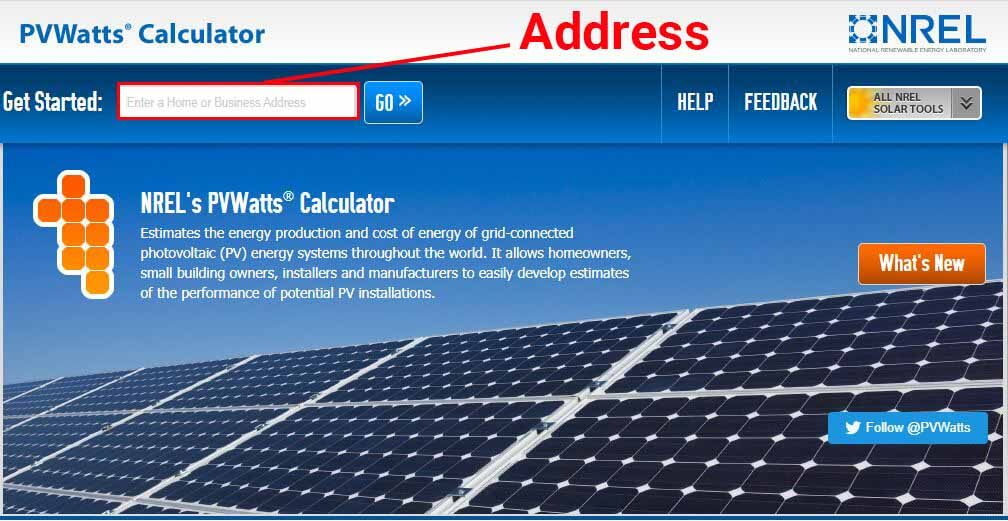# Can a 100 watt solar panel run a refrigerator?

A 100-watt solar panel can produce anywhere from 300Wh to 700Wh (Watt-hours) of energy in one day. At 12 Volts, and with an MPPT charge controller, that’s 25Ah to 60Ah (Amp-hours) of daily energy production. But is it enough to run a refrigerator?

In this article, I’ll show you how you can determine if 100 watts of solar is enough to run your refrigerator.

In case it is not enough, I’ll also show you how to calculate the amount of solar power that you need.## Can a 100 watt solar panel run a refrigerator?

Provided that there is a battery, an average amount of direct sunlight, and no partial shading, a 100 watt solar panel can definitely run a small (1.6-2.5 cubic feet) 12V car refrigerator, and can possibly run a 4.5 cubic feet mini-fridge.

However, bigger fridges will require more solar power to run without interruption.

For example, a standard 10 Cu. ft. RV fridge consumes about 1000Wh of energy per day. If the 100W solar panel is powering the fridge through a battery, it is possible to run the fridge for 8 to 16 hours per day.

To be 100% certain, you’ll need 2 estimates:

• The refrigerator’s daily energy consumption.
• The energy production of the solar panel in your location.

### How much energy does your refrigerator use?

The following table gives you an idea about the average energy consumption for different refrigerator sizes:

However, you should do your own estimations or measurements before making any decisions.

In general, there are 3 ways to determine how much energy your fridge consumes:

New refrigerators come with an EnergyGuide label that estimates their yearly energy consumption and how much it would cost to run them.

For example, the image below shows the EnergyGuide label of a 20.6 cubic feet refrigerator:The label estimates that this refrigerator uses 653 kWh (kilo-Watt-hours) per year. From this, we can calculate its estimated daily energy consumption:

Daily Energy Consumption (Wh) = 653 kWh ÷ 365

Daily Energy Consumption (Wh) = 1.789 kWh

Daily Energy Consumption (Wh) = 1789 Wh

For this particular example, the fridge should consume around 1800 Watt-hours per day give or take a 100Wh.

Now, what if you don’t have this label?

#### Use the manufacturers technical specifications:

On the spec sheet of the refrigerator, look for the wattage or the voltage/current.

For example, the image below shows the specification label of a 7 cubic feet refrigerator:The spec sheet shows 100W as the Rated Input. This means that the refrigerator uses 100 watts of power when it’s running.

However, sometimes the power consumption is not directly specified on the label. In other cases, the manufacturer specifies a few power inputs (Defrosting input, heater cord input, etc…), which makes it a little bit confusing.

To avoid confusion, you can use the voltage and the current to calculate the wattage:

Power (Watts) = Voltage (Volts) x Current (Amps)

For this example:

Power (Watts) = 220 V x 0.45 A

Power (Watts) = 100W

Still, this is not the energy consumption of the refrigerator, it’s the power it uses when the compressor is running.

However, we can still use the power usage to estimate the energy consumption:

Energy (Watt-hours) = Power (Watts) x Usage Time (Hours)

We have the power, but what is the usage time?

In general, the compressor has a duty cycle of about 0.33 (runs 33% of the time). This means that in 24 hours, the refrigerator is only on for about 8 hours.

Energy (Watt-hours) = Power (Watts) x Usage Time (Hours)

Energy (Watt-hours) = 100 Watts x 8 hours

Energy (Watt-hours) = 800 Wh

There are other factors that influence the duty cycle of the refrigerator, such as ambient temperature, how often the door is opened, how full the refrigerator is, etc…

To make sure you’re not underestimating its energy consumption, you can multiply the power usage by 10 or 12 instead of 8.

OR, you can go for a more precise method.

#### Use an energy measurement tool:

With tools such as the kill-a-watt or the Kuman meters, you can plug your fridge into the wall and monitor its energy usage over a certain period of time.

All you have to do is plug it in, wait 24 hours, and the monitor will give you the energy consumption in kWh (kilo-Watt-hours).

Now we need to look at the actual energy production of the 100 watt solar panel to see if it going to be enough.

### How much energy will you get from a 100W solar panel?

Since the power production of a solar panel fluctuates relative to how clear the sky is or how much direct sunlight it is receiving, the most precise way to measure its daily energy production is to use an electricity metering device.

However, thanks to the data collected by the National Renewable Energy Laboratory (NREL), anyone can estimate the amount of daily energy that their solar system should produce.

There are a few resources that give you the monthly or yearly average amount of sunlight that you receive daily in your location. The term for this is Peak Sun Hours.

For example, a location that receives an average 5kWh/m2 of sunlight per day can be said to receive 5 peak sun hours. You can then estimate how much energy a 100 watt solar would produce in this location:

Energy Production (Watt-hours per day) = Solar Panel Wattage (Watts) x Peak Sun Hours (Hours)

Energy Production (Watt-hours per day) = 100 W x 5 hours

Energy Production (Watt-hours per day) = 500 Wh/day

This means that in this location, the solar panel would produce around 500 Wh of energy per day. This is enough to run a 12 volt car refrigerator or even a mini fridge.

#### So, how many peak sun hours do you get?Then go to results.Based on your location, the tool gives you not only the yearly average, but also the monthly average peak sun hours, which is pretty useful.

For example, for the particular location that I used in the tool, a 100 watt panel would on average get 5.45 peak sun hours. Its average daily energy production can be calculated as such:

Energy Production (Watt-hours per day) = 100 W x 5.45 hours

Energy Production (Watt-hours per day) = 545 Wh/day

You can also estimate the energy production of the solar panel for a certain month.

For example, for this location, this is the daily energy production that should be expected in the month of July:

Energy Production (Watt-hours per day) = 100 W x 6.64 hours

Energy Production (Watt-hours per day) = 664 Wh/day

Now that you have an estimate of how much energy a 100 watt solar panel would produce in your location, compare it to the energy consumption of your refrigerator.

In case it is not enough, the next section explains how you can calculate the right amount of solar energy that is needed.

## How much solar power do I need to run a refrigerator?

To calculate the amount of solar power you need to run your refrigerator, divide the refrigerator’s estimated daily energy consumption by the number of peak sun hours in your location:

Solar power needed (Watts) = Estimated Daily Energy Consumption (Wh) ÷ Peak Sun Hours (hours)

For example:

Let’s consider a 20 cubic feet refrigerator that – on average – consumes 2000 Wh of energy per day. And let’s say the owner of this fridge lives in Tuscon, Arizona.

I used the PVWatts tool to check the amount of sunlight that this location receives, and it seems that on average, the location gets 6.54 Peak Sun Hours (receives 6.54kWh/m2 of sunlight per day):The solar power needed can be calculated as such:

Solar power needed (Watts) = Estimated Daily Energy Consumption (Wh) ÷ Peak Sun Hours (hours)

Solar power needed (Watts) = 2000 Wh ÷ 6.54 hours

Solar power needed (Watts) = 306 Watts

This means that the refrigerator in this example would need 306 watts of solar power to run.

However, it is better to use the peak sun hours from the month of December. This will ensure that the solar power is enough to run the refrigerator throughout the year.

The tool tells us that the location receives only 4.78 peak sun hours in December:

Solar power needed (Watts) = 2000 Wh ÷ 4.78 hours

Solar power needed (Watts) = 418 Watts

To run a refrigerator that consumes 2000Wh/day in Tuscon, the solar system would have to be rated at 418 watts or more.

But that’s not all, a solar energy system consists of more than just solar panels. You’ll also need :

• A battery bank: mainly to store the energy during the day and run the fridge during the night, but also to provide energy back-up.
• A solar charge controller: to maximize energy production and protect the solar panels and the battery.
• An inverter: if your refrigerator runs on AC power, an inverter will convert the DC power from the battery to AC.